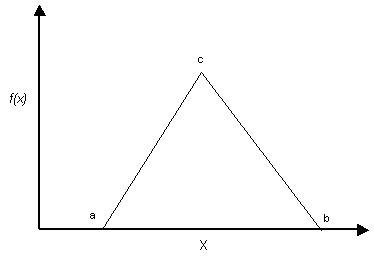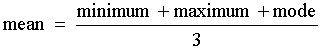RSWebinar: Designing Cost-Effective Ground Improvement Solutions with Settle3 | June 7th, 2023

# Triangular Distribution

You may wish to use a TRIANGULAR distribution in some cases, as a rough approximation to a random variable with an unknown distribution.

A TRIANGULAR distribution is specified by its minimum, maximum and mean values. It does not have to be symmetric, and can be skewed either to the left or right by entering a mean value greater than or less than the average of the minimum and maximum values.Triangular probability density function. Minimum = a, maximum = b, mode = c. For a symmetric distribution, mean = mode

### Note:

For a non-symmetric TRIANGULAR distribution, the mean value is not equal to the mode. The mode is the value of the variable at the peak of the TRIANGULAR distribution. The mean of a TRIANGULAR distribution has the following behaviour:

1. In general, the mean of a triangular distribution is always given by:2. If the distribution is symmetric, then the mean is equal to the mode.
3. For a left triangular distribution, the mode = minimum, and the mean = (2*minimum + maximum) / 3.
4. For a right triangular distribution, the mode = maximum, and the mean = (2*maximum + minimum) / 3.